Courses

Test: Name Reaction Level - 3

15 Questions MCQ Test Organic Chemistry | Test: Name Reaction Level - 3

Description
Attempt Test: Name Reaction Level - 3 | 15 questions in 30 minutes | Mock test for Chemistry preparation | Free important questions MCQ to study Organic Chemistry for Chemistry Exam | Download free PDF with solutions
*Multiple options can be correct
QUESTION: 1

The correct statement for osmium tetraoxide reagent is:

Solution:

A, B and C are correct. These are the properties of OSO4,

*Multiple options can be correct
QUESTION: 2

Solution:
QUESTION: 3

What is the product of the reaction between 1, 3-dibutene and bromoethane?

Solution:

Ans: a

Explanation: The electrons from one of the double bonds on the 1,3-dibutene create a new single bond. The other new single bond is created from the electrons in the double bond of the other reactant. These two new single bonds join the reactants to create a cyclic product.

The electrons from the other double bond in the 1,3-dibutene move between the carbon 2 and 3. Thus, the final product is a 6-carbon cycloalkene with a halogen substituent.

*Multiple options can be correct
QUESTION: 4

Among the following, the reagent(s) which is/are suitable for the following conversion:​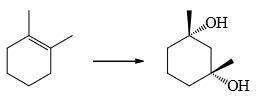Solution:
*Multiple options can be correct
QUESTION: 5

Which of the following combination(s) can be used to prepare alcohol given below: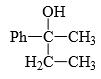Solution:

Option D produces 3-phenylpentan-3-ol while A, B, C produces our desired product.

*Multiple options can be correct
QUESTION: 6

Which of the following compound(s) is/are a suitable solvent for Grignard reaction:

Solution:
*Multiple options can be correct
QUESTION: 7

(Consider all step and intermediate) correct statement is/are: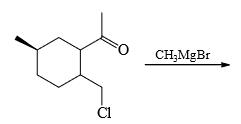Solution:

Since there is no hydrolysis happening, this is the final product.

Hence A, C and D are correct.

*Multiple options can be correct
QUESTION: 8

Choose the correct statement(s):

Solution:
*Multiple options can be correct
QUESTION: 9

Which of the following reagents give same product: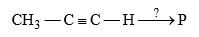Solution: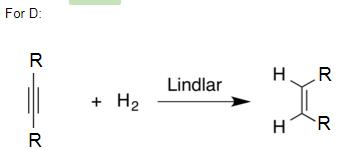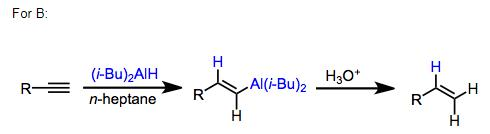Since they give the same product for these general reactions you can follow the same mechanism to get the required answer.

Hence B and D are the correct answers.

*Multiple options can be correct
QUESTION: 10

Which of the following reaction lead to increase in ring size in the product:

Solution:
*Multiple options can be correct
QUESTION: 11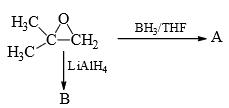Correct statement(s) is/are:

Solution:

Since LiAlH4 is stronger it will be able to reduce even the more hindered site and thus leaving the less hindered site for BH3/THF.

*Multiple options can be correct
QUESTION: 12

Which of the following reaction(s) is/are correct:

Solution:
*Multiple options can be correct
QUESTION: 13

Which of the following reaction(s) is/are correct:

Solution:
*Multiple options can be correct
QUESTION: 14

Which of the following is/are lead to ring formation:

Solution:
*Multiple options can be correct
QUESTION: 15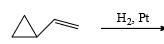Correct statement(s) is/are:

Solution:Use Code STAYHOME200 and get INR 200 additional OFF Use Coupon Code

Track your progress, build streaks, highlight & save important lessons and more!

Similar Content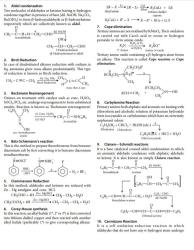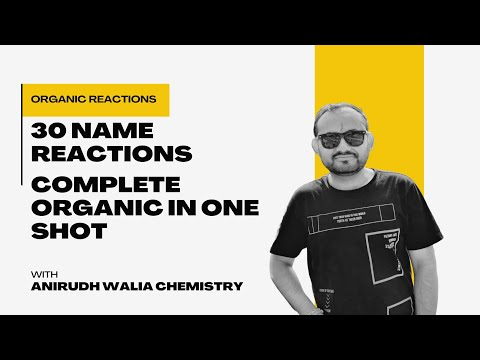Related tests